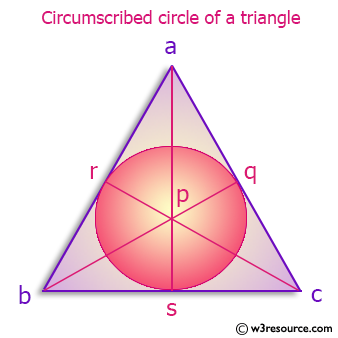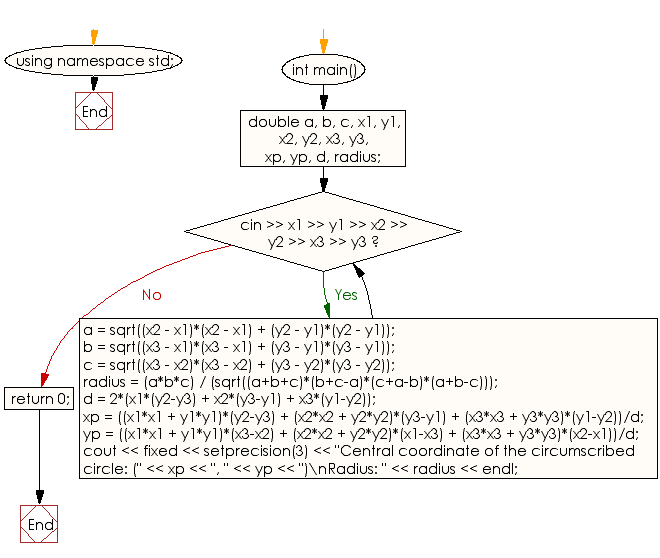﻿ C++ : Radius,coordinate - circumscribed circle of a triangle

# C++ Exercises: Prints the central coordinate and the radius of a circumscribed circle of a triangle

## C++ Basic: Exercise-67 with Solution

Write a C++ program that prints the central coordinate and the radius of a circumscribed circle of a triangle. This circle is created from three points on the plane surface.

Pictorial Presentation:Sample Solution:

C++ Code :

``````#include <iostream>
#include <iomanip>
#include <cmath>
using namespace std;
int main() {
double a, b, c, x1, y1, x2, y2, x3, y3, xp, yp, d, radius;
while (cin >> x1 >> y1 >> x2 >> y2 >> x3 >> y3) {
a = sqrt((x2 - x1)*(x2 - x1) + (y2 - y1)*(y2 - y1));
b = sqrt((x3 - x1)*(x3 - x1) + (y3 - y1)*(y3 - y1));
c = sqrt((x3 - x2)*(x3 - x2) + (y3 - y2)*(y3 - y2));
d = 2*(x1*(y2-y3) + x2*(y3-y1) + x3*(y1-y2));
xp = ((x1*x1 + y1*y1)*(y2-y3) + (x2*x2 + y2*y2)*(y3-y1) + (x3*x3 + y3*y3)*(y1-y2))/d;
yp = ((x1*x1 + y1*y1)*(x3-x2) + (x2*x2 + y2*y2)*(x1-x3) + (x3*x3 + y3*y3)*(x2-x1))/d;
cout << fixed << setprecision(3) << "Central coordinate of the circumscribed circle: (" << xp << ", " << yp << ")\nRadius: " << radius << endl;
}
return 0;
}
``````

Sample Output:

```Sample input : 6 9 7
Sample Output:
7 9 6
```

Flowchart:C++ Code Editor:

What is the difficulty level of this exercise?

﻿

## C++ Programming: Tips of the Day

Why is there no std::stou?

The most pat answer would be that the C library has no corresponding "strtou", and the C++11 string functions are all just thinly veiled wrappers around the C library functions: The std::sto* functions mirror strto*, and the std::to_string functions use sprintf.

Ref: https://bit.ly/3wtz2qA

We are closing our Disqus commenting system for some maintenanace issues. You may write to us at reach[at]yahoo[dot]com or visit us at Facebook